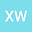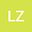Well-Conditioned Galerkin Spectral Method for Two-Sided Fractional Diffusion Equation with Drift and Fractional Laplacian
••• Xudong Wang,
• Lijing Zhao
Xudong Wang
Lanzhou University

Corresponding Author:xdwang14@lzu.edu.cn

Author ProfileLijing Zhao
Northwestern Polytechnical University
Author Profile## Abstract

###### Keywords:
Riemann-Liouville integral operator and Dirichlet boundary conditions and Galerkin spectral method and condition number
###### MSC:
26A33 and 34B60 and 65L60 and 65F35

In this paper, we focus on designing a well-conditioned Glarkin spectral methods for solving a two-sided fractional diffusion equations with drift, in which the fractional operators are defined neither in Riemann-Liouville nor Caputo sense, and its physical meaning is clear. Based on the image spaces of Riemann-Liouville fractional integral operators on $$L_{p}([a,b])$$ space discussed in our previous work, after a step by step deduction, three kinds of Galerkin spectral formulations are proposed, the final obtained corresponding scheme of which shows to be well-conditioned—the condition number of the stiff matrix can be reduced from $$O(N^{2\alpha})$$ to $$O(N^{\alpha})$$, where $$N$$ is the degree of the polynomials used in the approximation. Another point is that the obtained schemes can also be applied successfully to approximate fractional Laplacian with generalized homogeneous boundary conditions, whose fractional order $$\alpha\in(0,2)$$, not only having to be limited to $$\alpha\in(1,2)$$. Several numerical experiments demonstrate the effectiveness of the derived schemes. Besides, based on the numerical results, we can observe the behavior of mean first exit time, an interesting quantity that can provide us with a further understanding about the mechanism of abnormal diffusion.

17 Jan 2020Submitted to Mathematical Methods in the Applied Sciences
25 Jan 2020Submission Checks Completed
25 Jan 2020Assigned to Editor
25 Feb 2020Reviewer(s) Assigned
08 Sep 2020Review(s) Completed, Editorial Evaluation Pending
09 Sep 2020Editorial Decision: Revise Minor